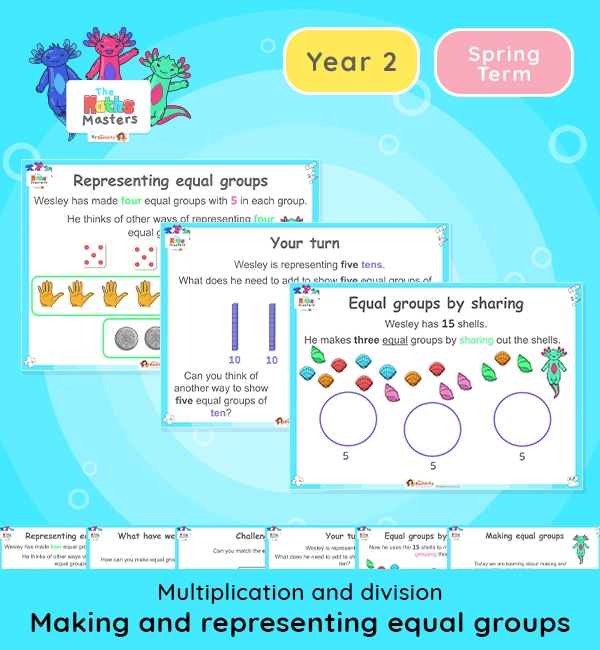# Year 2 | Making and Representing Equal Groups Lesson Presentation## White Rose Year 2 multiplication and division lesson plans

Aligned with the White Rose Maths scheme of work, this Year 2 | Making and Representing Equal Groups Lesson Presentation is fully editable, and is designed for the Year 2 maths curriculum covering the following maths objectives for the autumn term:

Topic: Multiplication and division

This is lesson focuses on making equal groups by grouping and sharing and representing equal groups in different ways. It builds upon the work on making equal groups from the end of the autumn term.

White Rose Maths Small Steps: Make equal groups

NC Links: Recall and use multiplication and division facts for the 2,5, and 10 times tables. *solve problems involving multiplication and division using materials, array, repeated addition, mental methods and multiplication and division facts including problems in context. *Count in steps of 2, 3, 5, and 10.

TAF Statements:– Recall and use multiplication and division facts for 2, 5, and 10 and use them to solve simple problems. Working At

Greater Depth – Use reasoning about number and relationships to solve more complex problems and explain their thinking.

Year 1 conceptual prerequisites: Count in multiples of 2, 5 and 10

## Recently Viewed